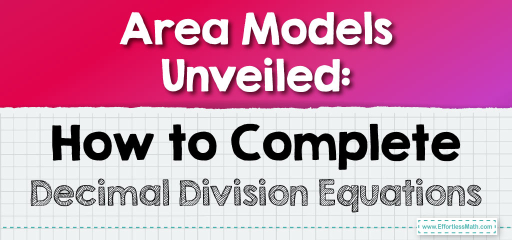# Area Models Unveiled: How to Complete Decimal Division Equations

Area models provide a visual representation of division, especially when dealing with decimals. By representing decimals as areas and using the model to perform division, we can easily complete division sentences. Let's explore this concept using area models.Visualizing with Area Models: Imagine a rectangle where the area represents the dividend and one of its sides represents the divisor. The length of the other side will represent the quotient.

## Dividing Decimals Using Area Models: Finish the Equation

### Example 1:

Complete the division sentence: $$0.6 \div \_ = 0.2$$.

Solution Process:

1. Visualize a rectangle with an area of $$0.6$$.

2. If one side of the rectangle is $$0.2$$, determine the length of the other side.

Using the area model, you can determine that the other side is $$3$$. Thus, $$0.6 \div 3 = 0.2$$.

The Absolute Best Book for 5th Grade Students

### Example 2:

Complete the division sentence: $$0.8 \div \_ = 0.4$$.

Solution Process:

1. Visualize a rectangle with an area of $$0.8$$.

2. If one side of the rectangle is $$0.4$$, determine the length of the other side.

Using the area model, you can determine that the other side is $$2$$. Thus, $$0.8 \div 2 = 0.4$$.

Using area models to visualize decimal division offers a clear and intuitive understanding of the process. It helps in breaking down complex divisions into simpler parts, making calculations more manageable. Whether you’re learning or teaching, area models serve as a powerful tool to grasp the intricacies of decimal division. Dive into the world of area models and watch the magic of division unfold before your eyes!

### Practice Questions:

1. Complete the division sentence: $$0.9 \div \_ = 0.3$$.

2. Determine the missing divisor: $$1.2 \div \_ = 0.4$$.

3. Complete the division sentence: $$0.5 \div \_ = 0.25$$.

4. Determine the missing divisor: $$0.7 \div \_ = 0.1$$.

5. Complete the division sentence: $$1.5 \div \_ = 0.5$$.

A Perfect Book for Grade 5 Math Word Problems!

1. $$3$$

2. $$3$$

3. $$2$$

4. $$7$$

5. $$3$$

The Best Math Books for Elementary Students

### What people say about "Area Models Unveiled: How to Complete Decimal Division Equations - Effortless Math: We Help Students Learn to LOVE Mathematics"?

No one replied yet.

X
51% OFF

Limited time only!

Save Over 51%

SAVE $15 It was$29.99 now it is \$14.99